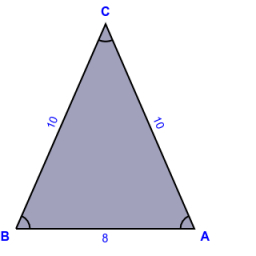# Iso triangle

An isosceles triangle with a base of 8 cm. Its circumference is 28 cm. Calculate the height of the triangle.

h =  9.1652 cm

### Step-by-step explanation:

Try calculation via our triangle calculator.Did you find an error or inaccuracy? Feel free to write us. Thank you!

Tips to related online calculators
Pythagorean theorem is the base for the right triangle calculator.